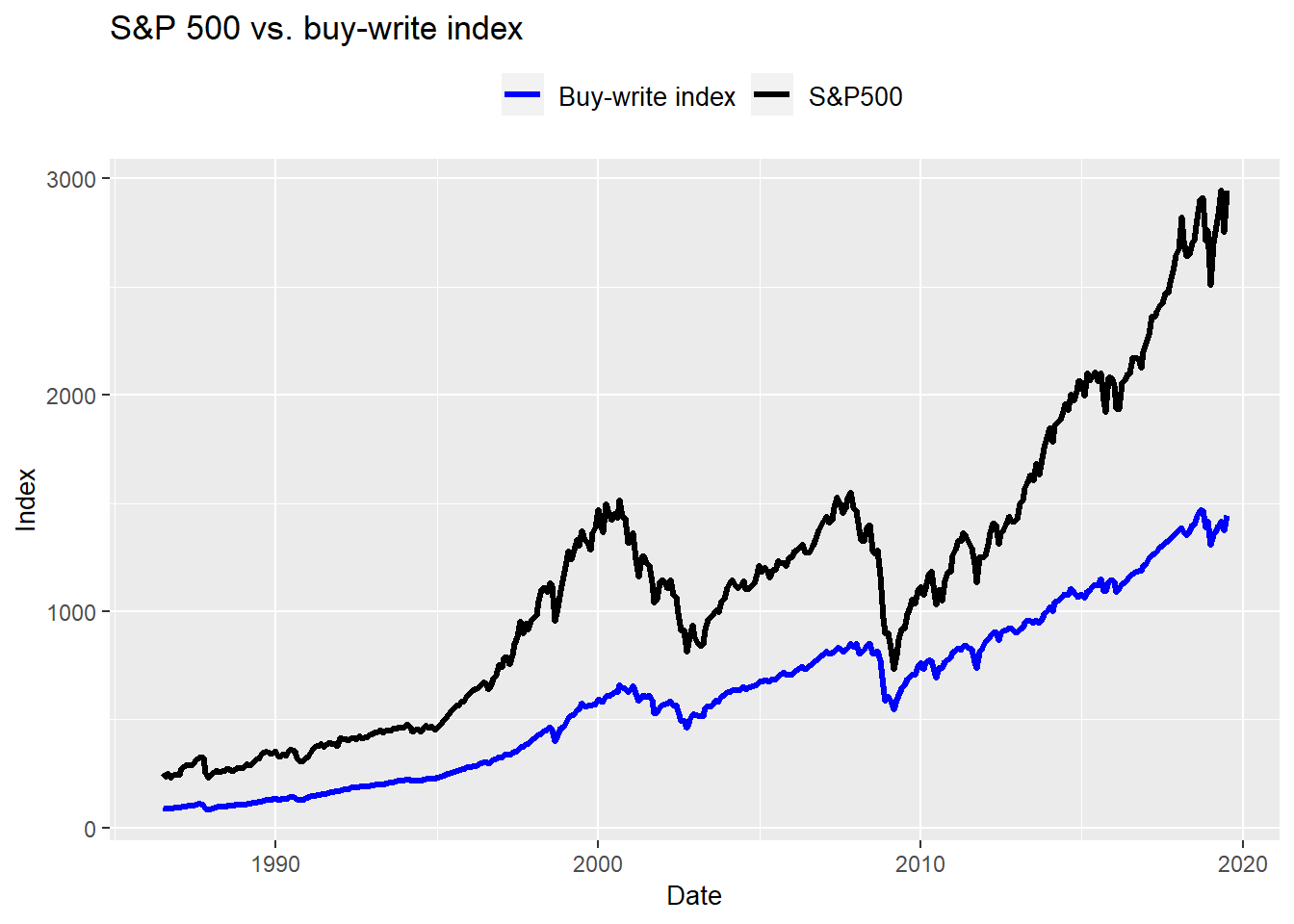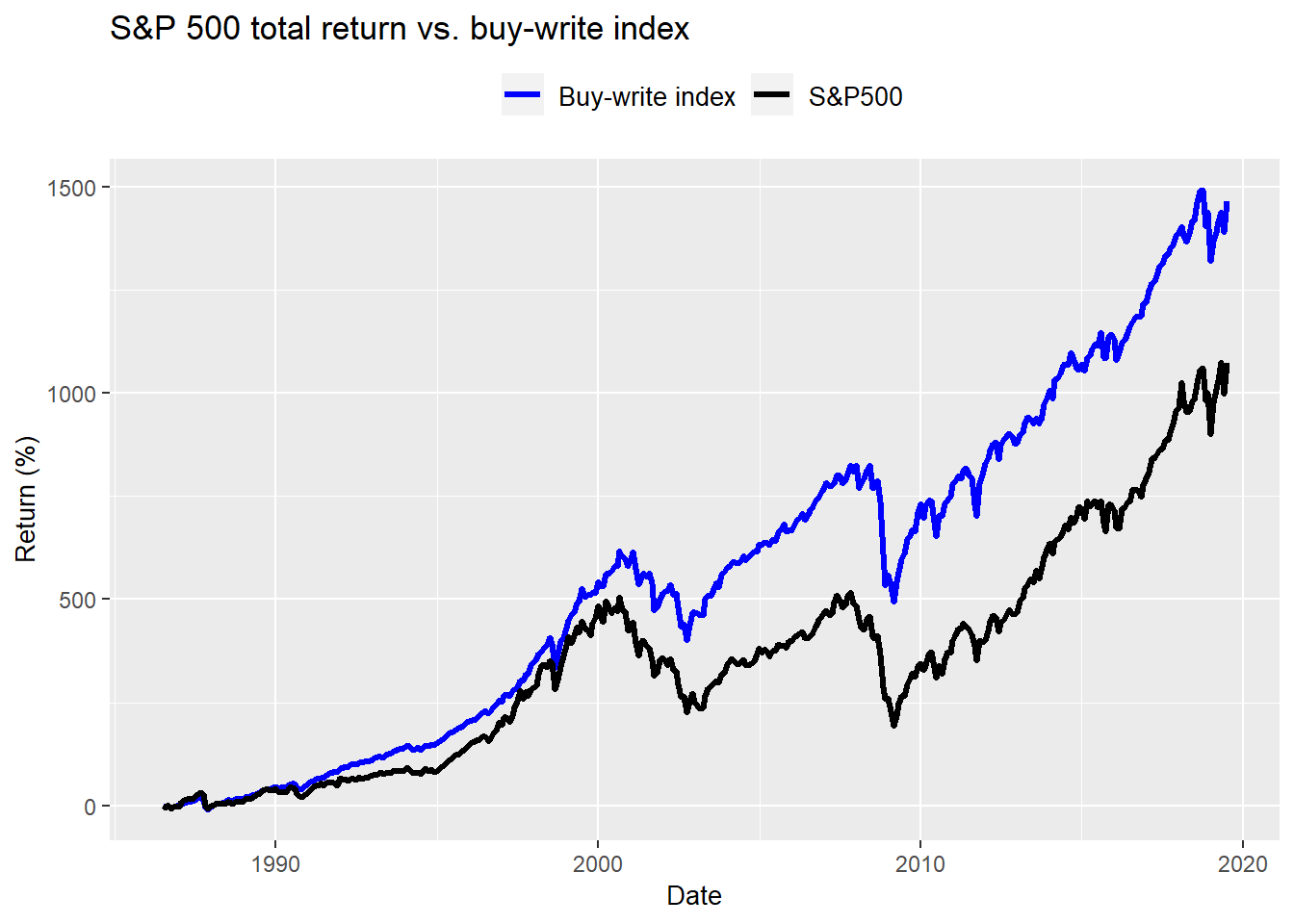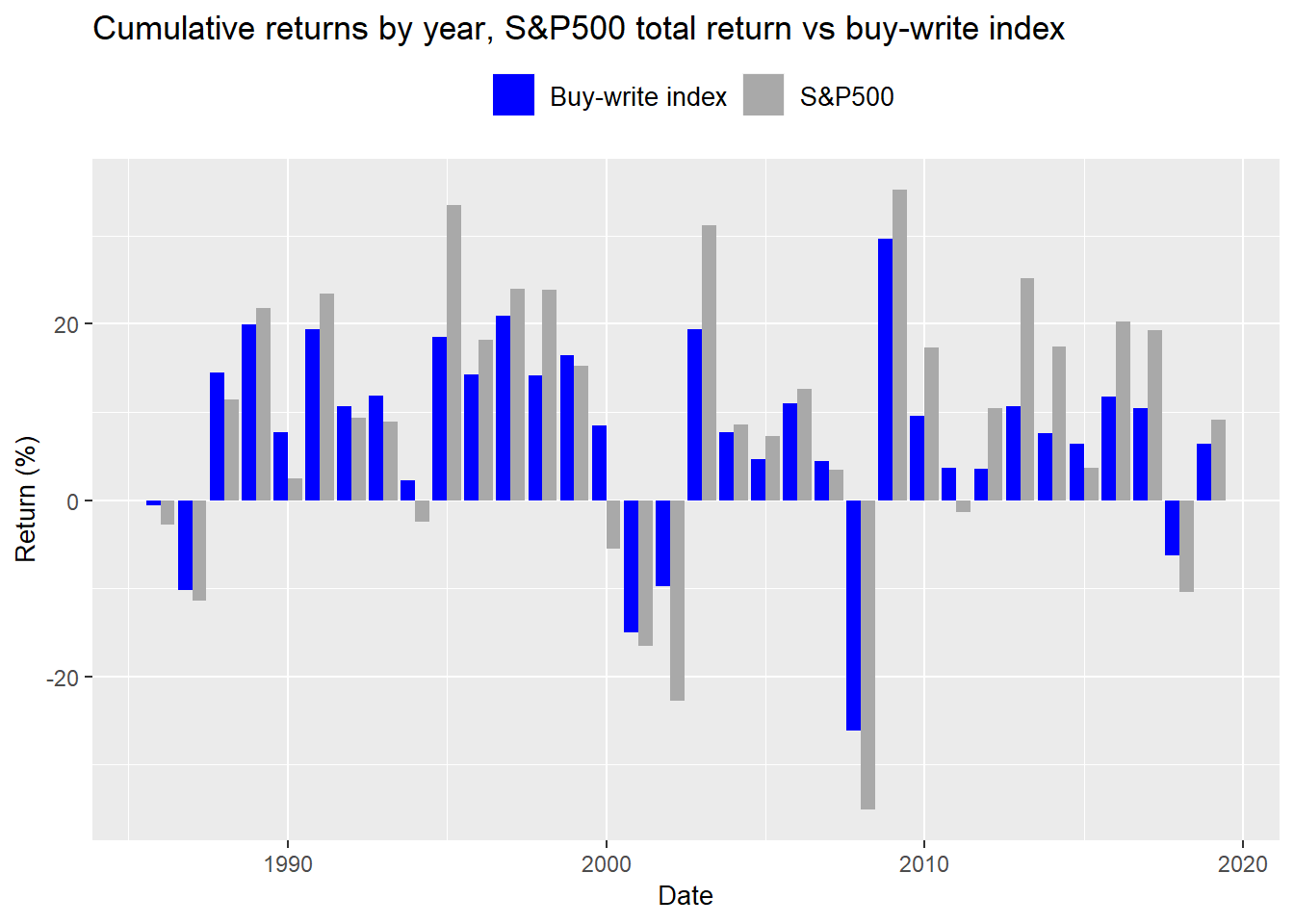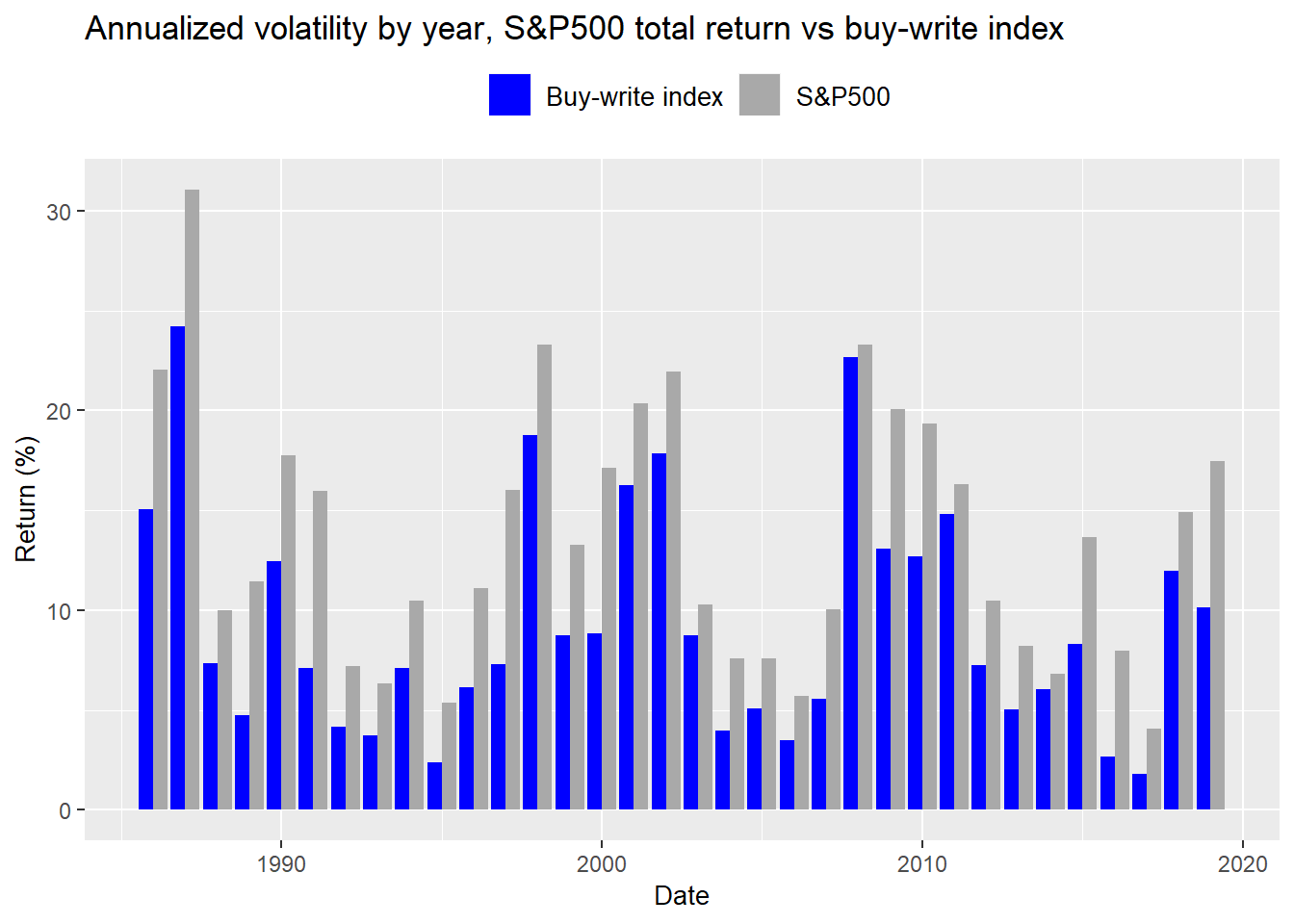# Who's covered

One of the simplest options strategies is known as the covered call. For this strategy, an investor who already owns a stock elects to sell (or write) an option contract to surrender that stock at a specified price (known as the strike) at some point in the future (also known as expiration). The sale of the contract generates income for the investor, not unlike when an insurance company receives premiums from selling an insurance contract.

It is called “covered” because the investor owns the stock, as opposed to “naked” when the investor does not, and thus has the capacity to deliver the stock should the contract be exercised. The strategy is also often referred to as “buy-write” in that the investor buys the underlying stock while simultaneously selling a call option in one transaction. If the investor does not own the stock, he or she will execute a buy-write. But if the investor already owns the stock, he or she will execute a covered call.

In terms of risk/reward, the maximum loss on the covered call strategy is the amount paid for the asset less any premiums received from selling the contract. The maximum gain is the difference between the strike price of the option contract and the amount paid for the stock plus premiums received. In effect, the investor is willing to cap his upside potential in return for receiving a guaranteed premium.

Why would anyone want to cap his upside while only modestly improving his downside? For one, most stocks are unlikely to go to zero unless they’re Enron or Theranos. Similarly, most stocks don’t move up in a straight line steadily over time, so there is a chance that, like insurance, the event that would force the investor to surrender the stock never occurs, allowing him or her to pocket the premium. Additionally, depending on how far away the strike is placed relative to the stock price, the likelihood the stock could get “called away” (a contractually obligated surrender) declines at an accelerating rate. Throw in the time factor and writing covered calls looks very similar to writing insurance premiums.

From an investor perspective, then, writing covered calls is often considered a yield-enhancing strategy. For example, say the annual expected price return on a stock is 7% with an annualized standard deviation of 12%. If the investor could sell a call that expires one-year hence, at 12% above the current stock price, and receive a credit from the sale equivalent to a 1% of the current stock price, then the investor theoretically has a 70% chance of improving his return to 8%. But, that’s the most the stock can return.

Alternatively, the investor could sell calls that expire sooner but are less than 12% away since the range of probable moves in a less than 12-month period would be less than 12%. Say the investor sells calls that expire in a month that are 3% above the current stock price and yield 0.5% in premium. That investor could generate an additional 6% in yield on an annual basis!

Unfortunately, things don’t work that way. Nonetheless, covered call writing is a popular strategy. Popular enough that the Chicago Board of Options (CBOE) publishes a “buy-write” index, known as the “BXM”, on the strategy. It also publishes other indices, all of which provide us with a treasure trove of data to analyze. In the ensuing posts, we’ll look at a number of these strategies.

In this post we’ll begin looking at the buy-write or BXM index. Later, we’ll examine some of the findings from pubished reports on the covered call strategy. You can find information on the CBOE buy-write strategy here and some of the studies we’ll look at here and here.

## Call to cover

To begin let’s look at the index and execute some exploratory data analysis. Here’s a graph of the S&P500 index vs. the CBOE buy-write index. We’ll be using BXM or buy-write interchangeably.A couple points worth noting is that the indices don’t start on the same basis, and, the buy-write index includes dividends. So we should normalize this and compare the buy-write to the S&P500 total return index. Doing all that we arrive at the following.Wow! What a difference scaling makes. Almost makes us want to go out and invest right now. But wait. It’s an index so it isn’t investable.

A couple of things that first jump out. The shape of both curves is relatively the same, as would be expected since the BXM is based on the S&P. Some of the outperformance seems to be weighted toward the beginning of the time series. It’s also not so easy to tell whether the BXM is more or less volatile from this long series. So we’ll have to look at those points individually.

Here’s a yearly bar chart of cumulative returnsWhat should be readily apparent is the BXM doesn’t seem to outperform the S&P too much. When it does, the S&P is usually having a bad year. Furthermore, when the S&P outperforms the BXM, it does so by a great deal, usually on the positive side. In total, BXM only outperformed the S&P 16 times out of 34 for the years we have data — 1986-2019.

If the BXM seems to underperform only modestly on a yearly basis, what causes the cumulative outperformance? The nature of returns. The BXM generally outperforms when the S&P suffers a down year. By experiencing less severe declines in a few bad years, the BXM has less ground to make up to get back to flat. When the S&P lost 35% in 2008, the BXM only lost 26%. Even though the BXM did not rebound as much in 2009, it was sufficient to keep it ahead of the S&P for that year. The table below shows the average returns of the BXM and the S&P 500 when the BXM’s return exceeds or trails the S&P 500

Table 1: Average over and underperformance of the buy-write index relative to the S&P500 total return
Performance BXM return (%) S&P 500 return(%)
Outperformance 1.1 -3.4
Underperformance 13.3 19.9

Another reason that the covered-calls attract investors is the assumption that it lowers volatility. Why would this be the case? Since one collects some cash from selling the call, the downside is less severe while the upside is, of course, capped. Hence, in any one year the covered-call strategy is likely to see both higher lows and lower highs than simply owning the stock. That narrowed range of outcomes means volatility (the traditional measure of risk) is lower, hence risk-adjusted returns are better. Let’s see if that holds based on the data. In the bar chart below we present the annualized volatility by year for the buy-write index and the S&P 500.Apparently, this assumption holds because there appears to be no year in which the BXM is more volatile than the S&P. But that lower volatility does come at price, since, as we noted, the upside is capped. Whether an investor is being adequately compensated for limiting his upside potential or whether this narrowe range of outcomes is an appropriate measure of risk will be left for later discussions.

In our next post, we’ll examine some claims made by one report on the buy-write strategy; namely, that it was found to have higher growth, lower volatility, and better risk-adjusted returns. Until then, here is the underlying code:

# Load package
library(tidyquant)

# Get data and process

cboe <- cboe %>%
rename("date" = X__1,
"BXM" = BXMSM,
"BXY" = BXYSM,
"CLL" = CLL*,
"VIX" = VIX®) %>%
mutate(date = ymd(date))

colnames(cboe) <- tolower(colnames(cboe))

# Graph data

# Graph indices
cboe %>%
gather(key, value, -date) %>%
filter(key %in% c("bxm", "spx")) %>%
ggplot(aes(date, value, color = key)) +
geom_line(lwd = 1.1) +
scale_color_manual("", labels = c("Buy-write index", "S&P500"),
values = c("blue", "black"))+
theme(legend.position = "top",
legend.text = element_text(size = 10),
axis.title = element_text(size = 10)) +
labs(x = "Date",
y = "Index",
title = "S&P 500 vs. buy-write index")

# Graph return of buy-write vs S&P
cboe %>%
gather(key, value, -date) %>%
# mutate(value = as.numeric(value)) %>%
filter(key %in% c("bxm", "spx")) %>%
group_by(key) %>%
mutate(value = (value/value-1)*100) %>%
ggplot(aes(date, value, color = key)) +
geom_line(lwd = 1.1) +
scale_color_manual("", labels = c("Buy-write index", "S&P500"),
values = c("blue", "black"))+
theme(legend.position = "top",
legend.text = element_text(size = 10),
axis.title = element_text(size = 10)) +
labs(x = "Date",
y = "Return (%)",
title = "S&P 500 total return vs. buy-write index")

# Yearly returns
cboe %>%
gather(key, value, -date) %>%
filter(key %in% c("bxm", "sptr")) %>%
mutate(year = year(date)) %>%
group_by(key, year) %>%
mutate(value = ROC(value)) %>%
summarise(value = Return.cumulative(value)) %>%
ggplot(aes(year, value*100, fill = key)) +
geom_bar(stat = "identity", position = "dodge") +
scale_fill_manual("", labels = c("Buy-write index", "S&P500"),
values = c("blue", "darkgrey"))+
theme(legend.position = "top",
legend.text = element_text(size = 10),
axis.title = element_text(size = 10)) +
labs(x = "Date",
y = "Return (%)",
title = "Cumulative returns by year, S&P500 total return vs buy-write index")

# How many times does bxm outperform
perf <- cboe %>%
gather(key, value, -date) %>%
filter(key %in% c("bxm", "sptr")) %>%
mutate(year = year(date)) %>%
group_by(key, year) %>%
mutate(value = ROC(value)) %>%
summarise(value = Return.cumulative(value)) %>%
count(bxm > sptr)

# Average returns
cboe %>%
gather(key, value, -date) %>%
filter(key %in% c("bxm", "sptr")) %>%
mutate(year = year(date)) %>%
group_by(key, year) %>%
mutate(value = ROC(value)) %>%
summarise(value = Return.cumulative(value)) %>%
mutate(perf = bxm - sptr,
opf = ifelse(perf > 0, "Outperformance", "Underformance")) %>%
group_by(opf) %>%
summarise(bxm_ret = round(mean(bxm),3)*100,
sptr_ret = round(mean(sptr),3)*100) %>%
rename("Performance" = opf,
"BXM return (%)" = bxm_ret,
"S&P 500 return(%)" = sptr_ret) %>%
knitr::kable(caption = "Average over and underperformance of the buy-write index
relative to the S&P500 total return")

# Volatility by year
cboe %>%
gather(key, value, -date) %>%
filter(key %in% c("bxm", "sptr")) %>%
mutate(year = year(date)) %>%
group_by(key, year) %>%
mutate(value = ROC(value)) %>%
summarise(vol = sd(value, na.rm = TRUE)*sqrt(12)*100) %>%
ggplot(aes(year, vol, fill = key)) +
geom_bar(stat = "identity", position = "dodge") +
scale_fill_manual("", labels = c("Buy-write index", "S&P500"),
values = c("blue", "darkgrey"))+
theme(legend.position = "top",
legend.text = element_text(size = 10),
axis.title = element_text(size = 10)) +
labs(x = "Date",
y = "Return (%)",
title = "Annualized volatility by year, S&P500 total return vs buy-write index")